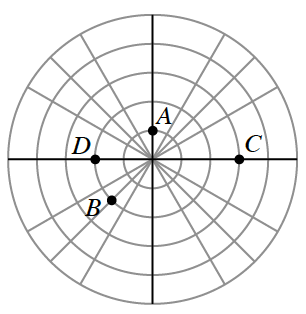### Home > PC3 > Chapter 11 > Lesson 11.1.2 > Problem11-31

11-31.In the figure at the right, there are points marked on the polar grid. What are the polar coordinates of each of those points?

Polar coordinates are of the form (radius, angle).
For example, point $B$ has a radius of $2$, so its coordinates are $(2, \text{angle})$.
Also, point $D$ is at an angle of $π$, so its coordinates are $(?, π)$.